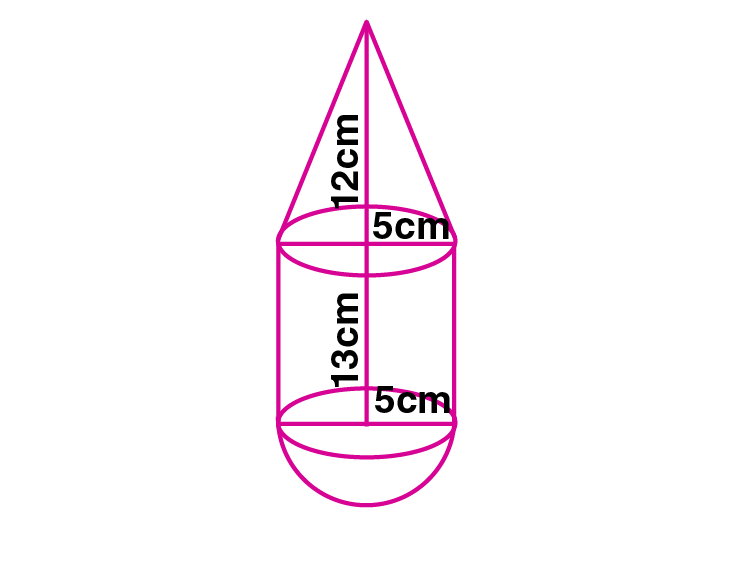Newbie

# A toy is in the shape of a right circular cylinder with a hemisphere on one end and a cone on the other. The height and radius of the cylindrical part are 13 cm and 5 cm respectively. The radii of the hemispherical and conical parts are the same as that of the cylindrical part. Calculate the surface area of the toy if the height of the conical part is 12 cm.

• 0

This is the Important question of class 10 Based on Mensuration Chapter of M.L Aggarwal book for ICSE BOARD.
A toy is in the shape of a right circular cylinder with a hemisphere on one end and a cone on the other.
The height and radius of the cylindrical part are given. The radii of the hemispherical and conical parts are the same as that of the cylindrical part. Solve this question.
This is the Question Number 21, Exercise 17.4 of M.L Aggarwal.

Share

1.Given height of the cylinder, H = 13 cm

Radius of the cylinder, r = 5 cm

Radius of the hemisphere, r = 5 cm

Height of the cone, h = 12 cm

Radius of the cone, r = 5 cm

Slant height of the cone, l = √(h2+r2)

= √(12)2+(5)2)

= √(144+25)

= √169

= 13 cm

Surface area of the toy = curved surface area of cylinder +curved surface area of hemisphere+ curved surface area of cone

= 2rH+2r2+rl

= r(2H+2r+l)

= (22/7)×5(2×13+2×5+13)

= (110/7)×(26+10+13)

= (110/7)×49

= 110×7

= 770 cm2

Hence the surface area of the toy is 770 cm2.

• 0